## 2.2.1 Ab-initio calculation of magnetic properties.

Spinand orbitalmoments, its coupling and the exchange interaction are pure quantum phenomena responsible for the ferromagnetism. With simple quantum statistical models we can explain some observed effects as the magnetocrystalline anisotropytemperature dependence exponent [Callen 66], where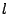is the order of the spherical harmonics describing the angular dependence of the local anisotropy, known as Callen-Callen or Akulov law. Another example is the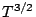temperature dependence of magnetization deviation from itsvalue or Bloch law [Bloch 30]. However, analytical models are not able to treat complex systems and to extract the intrinsic parameters of magnetic materials. In the mentioned above example of the magnetic anisotropy temperature dependence the analytical calculation is not able to provide the zero temperature value, needed in order to complete the whole temperature dependence. First principles numerical calculations are the tool needed to provide the strength of the interactions and the structure of the material at the atomic level. Unfortunately, the full Hamiltonian eigenvalue problem is still only solvable in few cases and extra approximations are needed. The first of them is the Born-Oppenheimer approximation in which only the electronic degrees of freedom are taken into account quantically and the nuclei move in the resulting ionic potential. Another very useful approximation is to consider only the outer electrons and to treat the inner electrons as pseudopotential acting on the outer electrons. A powerful and amply used tool is found in the density functional theory (DFT) which has its origin in the Hohenberg-Kohn Theorem that transforms the complex many-body problem of interacting electrons and nuclei into a coupled set of one-particle Kohn-Sham equations. The problem changes from a eigenvalue problem to a variational problem, which is computationally much more manageable. The energy becomes a functional of the electronic density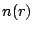. The exact functional is not known, because the exchange and correlation terms are not, and additional approximation have to be invoked: Local Density Approximation(LDA), General Gradient Approximation(GGA) , etc.

In the magnetic systems variations have to be included: our scheme has to be spin aware (instead of LDA the local spin density(LSDA) is used). The relativistic spin-orbit coupling has to be added to the functional in order to calculate the magnetocrystalline anisotropy. This term is very weak and can be considered as a perturbation but provokes the need to change the representation fromto the total angular moment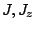becauseandare no longer good quantum numbers.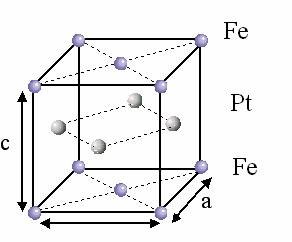An example of ab-initio calculations is the work on the magnetic anisotropy of FePt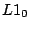[Mryasov 05], which is one of the principle materials under investigation in this thesis. In what follows, we will use the FePt parameters, estimated in this work. The high anisotropy fct FePtphase consists of alternated atomic layers of Fe and Pt (see Fig. 2.2). The magntetocristallyne anisotropy is mainly due to the contribution from the 5d element (Pt) having large spin-orbit coupling whereas the 3d element (Fe) provides the large magnetic moment. The work uses the constrained LSDA (CLSDA) method, which allows to treat non-collinear magnetic order [Dederichs 84]. The Pt, normally non-magnetic, has an induced non-localized magnetic moment (see Fig. 2.3) proportional to the exchange field from the Fe moments. Treating the Pt moments as independent degrees of freedom gives incorrect results (low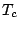and `soft' Pt layers). The exchange of the Fe sites is not limited to nearest neighbors and can be ferromagnetic (positive) or antiferromagnetic (negative) (see Fig. 2.4). Based on those facts and the ab-initio calculated parameters, the authors of Ref. [Mryasov 05] introduced an effective hamiltonian that includes only the Fe magnetic moment degrees of freedom:(2.1)

whereis the single ion anisotropy,is a new two ion anisotropy andis the effective exchange interaction parameter. Here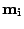denote Fe sublattice spin moments which can be treated as unit vector as will be explained in the next subsection. The contribution of the Pt moments is the new effective parameters for the exchange and the single ion anisotropy and a new term in the hamiltonian: the two ion anisotropy. The result of this mechanism is a large uniaxial out-of-plane anisotropy and the authors obtained a bulk value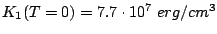.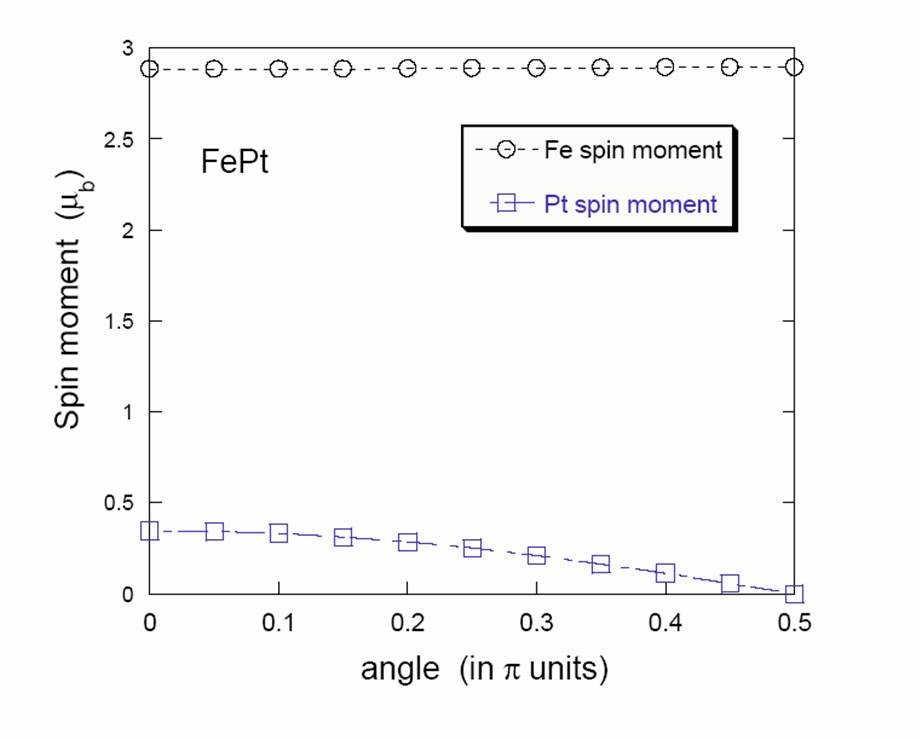2008-04-04